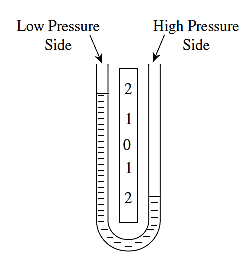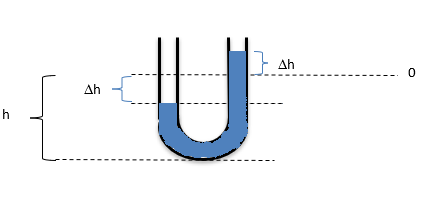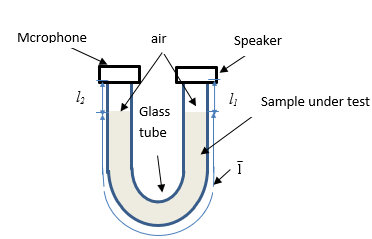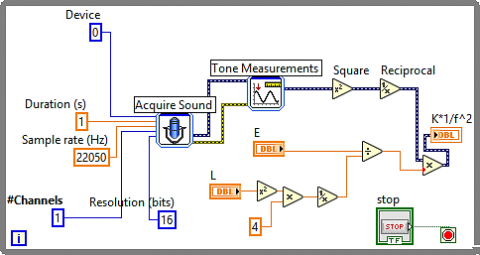# U-shape Acoustic Liquid Densitometer

U-shape Acoustic Liquid Densitometer

Tariq M. YounesMohammad A. AlKhedher Kalil Abu Shgier Farouq Al Taweel

Al Balqa Applied University, Faculty of Engineering Technology, Mechatronics Engineering Department, Amman, Jordan

Abu Dhabi University, Mechanical Engineering Department, Abu Dhabi, UAE

Al Balqa Applied University, Faculty of Engineering Technology, Mechanical Engineering Department, Amman, Jordan

Al Balqa Applied University, Faculty of Engineering Technology, Communication Technology Department, Amman, Jordan

Corresponding Author Email:
tariqmog@bau.edu.jo
Page:
123-128
|
DOI:
https://doi.org/10.18280/i2m.180205
16 January 2019
|
Accepted:
1 April 2019
|
Published:
30 June 2019
| Citation

OPEN ACCESS

Abstract:

The growing interest in measuring density of liquids has led to the need to develop new types of densitometers that can be used in several applications. The purpose of this work is to propose a simple densitometer based on measuring the frequency of created standing wave with a tube filled by a liquid under test. The developed approach is utilized to obtain a relationship between the density of some liquids and the frequency. The data acquisition and signal processing are carried out by LabVIEW software. It was shown that is a clear relationship between the frequency of standing wave created within a resonance tube in form of U-shape and the density of tested sample. This result can assist in production of cost-effective and portable densitometers.

Keywords:

acoustic, densitometer, hydrostatic, resonance, standing wave

1. Introduction

The safety of human being in modern industrial areas depends on the quality control of objects functioning in most industries such as: vehicles, machinery, transport and other means which characterized by using various types of fluids such as fuel, lubricants, various oils, solvents, etc.  The quality control of these fluids is defined and featured utilizing several measurement and instrumentation means which practically investigate several physical and chemical parameters related with these fluids .  Since   the reliable operation of machines and mechanisms   practically depends on status of these fluids, in industrial applications, the status of the fluid is gauged by comparing its current physical, chemical, and other parameters with their preset values . Although, the existed measurement and instrumentation techniques are capable to be used to measure several fluid parameters by the help of various types of transducers, the metrological requirements applied in process control significantly reduces many kinds of these transducers due to the environmental condition where the measurements are applied such as: high temperature, aggression and/or toxicity of the test objects .

In particular, the operation of nuclear power plants (controlling the liquid wastes), in military and civil aviation and road transport (quality control of fuels and lubricants), food industry (milk production, syrups and other liquids) a widely range of methods and means are used to measure viscosity and density of liquids or liquefied media. These include various types of viscometers (capillary, rotational and vibrational) densitometer (float, mass, hydrostatic and vibration, radiation and ultrasonic) [4-5].

The density measurement is carried out for identification of liquids and quality control, as well as for performing the management of the quantities of raw materials, fuels, reagents and finished products.

In practical application several types of densitometer are used such as: floating, mass and hydrostatic densitometers. Although, these densitometers are easy to operate, but almost impossible to automate .

One of the most popular densitometers used in practice is radioisotope densitometer . The operation principle of this densitometer is based on the determination of the attenuation of the radiation beam as a function of its absorption or scattering by a layer of liquid that is related to the density of the tested medium. Their advantage is the lack of contact with the liquid being investigated, and the disadvantage is the need to protect the personnel .

As long experience shows, in many industries the use of ultrasonic transducers as a measuring device for quality control of liquids and other products is quite effective [9-10]. Currently, the measurement of speed of sound is widely used to measure the properties of liquid .

These methods included the use of complicated, costly or dangerous materials or equipment. The purpose of this paper is to overcome these challenges to introduce a simple yet effective method of density measurement.

This paper establishes a prototype to measure liquid characteristics based on the operation principle of ultrasonic method of measuring the functional dependence of the parameters of ultrasonic waves propagating in a liquid (velocity of propagation, attenuation coefficient, longitudinal and shear acoustic impedances) on the properties of the investigated liquid.

The remainder of this paper is organized as follows: Literature review section introduces recent work in the field of study, Theoretical background section describes the scientific fundamentals of the proposed method, Methodology section summaries the procedure followed in this work, Experimental setup section explain the prototype manufactured and measurement method, Software design and implementation section details signal acquiring and processing and Experimental results and data analyses section discusses different measurements achieved for different cases that validates the methodology followed in this paper.

2. Literature Review

Density of a substance is called a physical quantity that determines the mass contained in unit volume . Therefore, it is calculated as the ratio of the mass of a substance to the volume it occupies. Measuring devices used to measure density are called density meters or densitometers. In general, there are two approaches utilized to measure density of liquids. The first one is widely used in industry, laboratories and it based on measurement of the mass of liquid with known volume. In this case the mass (with a known volume) of liquid is determined directly using standard calibrated weighting device or indirectly by measuring other physical quantities have a known functional relationship with the mass of the liquid. According to the operation principle, these densitometers are classified into float, mass, hydrostatic and vibration densitometers.

The operation principle of float or aerometric densitometers based on Archimedes principle which states that the weight of liquid displaced by a floating hydrometer equal to its mass. In mass densitometer (pycnometer) the density is determined by measuring the ratio between the mass of liquid and to its volume .

Hydrostatic densitometers are capable to measure density by measuring the pressure of the liquid column of constant height. The density has a linear relationship with the measured hydrostatic pressure [14-15]. The operation principle of vibrating densitometers is based on measuring the resonance frequency (excited by a vibration source -transmitter) which is a function of the density of the tested liquid . Since the oscillation frequency can be measured with high accuracy, the error in measurement is sufficiently small, not exceeding a few hundredths of a percent. The sensing element typically is a polished inside a metal tube in a U- shape. U-Shape tube is a special device pressed against the tube from outside and causing oscillatory motion at the resonant frequency. While the tube is not filled by a liquid, the measured resonance frequency is w0 . When the tube is then placed directly in the stream of the analyzed fluid, causing its oscillation w frequency is changed.

In the second approach, awareness about the volume value of the liquid is not necessary while one or a number of physical parameters characterized the liquid (functionally directly related to density) are measured.  For example, density meters that use radioactive and ultrasonic are used to measure the density of liquid by measuring liquid one or more liquid properties. According to the operation principle, these densitometers use radioactive and ultrasonic radiations to measure density .

The operation principle of radioisotope densitometers is based on detection of attenuation of the radiation beam as a result of absorption or scattering by the liquid layer . The attenuation of radiation is associated functionally with the density of the medium. The most common densitometers use γ-radiation. In such a device the radiation from a source (60Co, Cs) passes through the liquid layer in the vessel and reach the receiver. The receiver signal which is a function of the measured density is amplified and converted into electrical signal .

In ultrasonic or even sound technique used to measure the density of the liquid, all measured parameters of longitudinal ultrasonic/ sound waves are characterized by their distribution, which depends on the density of measured: the wave resistance of the liquid, the speed or velocity of sound in the media and absorption coefficient .

Acoustic detector for measuring density of vapors of a liquid is also presented in work . The proposed technique is based gas chromatography analyses and measuring the speed of sound in a resonance tube which is a part of a chromatograph. The measurement of the resonance frequency is a function of the density of a liquid. Experience have shown that acoustic methods are widely used as primary technique for monitoring the quality of liquids prove to be quite effective in numerous industries.

Industrial application where acoustic measurement is used to determine the characteristics of liquid media base on the functional dependence of the parameters of acoustic waves propagating in liquids (propagation velocity, attenuation coefficient, longitudinal and shear acoustic impedances, frequency) and fluid properties. Therefore, the process of developing new methods and techniques for measuring density of liquid media using acoustic waves is still continuous process. In spite of the benefits presented use acoustic methods and techniques for density measurement in many literatures, they are restricted in its complexities [23-25]. The methods and techniques require the use special data logging, signal conditioning and other measurement chains. In this work, a simple acoustic method for measuring the density of liquid is presented. The method based on measuring the frequency of transmitted acoustic signal within a U-shape tube filled by the liquid sample. In work, it was shown   waves can be transmitted within a tube filled by a liquid or gas under the following three conditions [15, 22]:

-  When a free mode of resonance is applied in measurement

-  When a forced mode of resonance is applied in measurement and an acoustic flow is not presented

-  When an acoustic standing wave is created, and an acoustic flow is presented.

3. Theoretical Background

The U-shapes tube is widely used in measurement . One of the most popular measurement where the U-shape is used is pressure measurement. It is the liquid manometer consisting of communicating vessels in which the measured pressure is determined by one or several levels of liquid. In U-shaped glass manometers the free end of a tube is reported with the atmosphere, and the measured pressure is brought to another end. The simplest U-Shape tube used for pressure measurement is shown in Figure 1 .

## f1.pngFigure 1. Simple U-Shape tube used for pressure measurement

The difference between the two columns of liquid is an indication of the pressure differential. The principle of measurement is based by comparing the pressure p being measured with the height h of a liquid column using the law

$\Delta \mathrm{p}=\Delta \mathrm{h} \cdot \rho_{\mathrm{m}} \cdot \mathrm{g}$   (1)

The literature review also shows that the U-shape tube is used to measure density. This device is known as vibrating U-tube or Oscillating U-tube . The operation principle of the oscillating U shape densitometer is based on the law of harmonic oscillation. The electromagnetic force is subjected to a sample to be analyzed. The density is determined by measuring the frequency and duration of vibration of the tube filled with the sample.

## f2.pngFigure 2. Unbalanced U-Shape tube showing column height

As shown in Figure 2, when the liquid in both bends is unbalanced, there is a returning force acting on all liquid on cross section S:

$\mathrm{F}=\rho \mathrm{gS} \times 2 \Delta \mathrm{h}$   (2)

The acceleration of motion is found by Newton's second law

$\mathrm{a}=\mathrm{F} / \mathrm{m}=-\rho \mathrm{gS} \times 2 \Delta \mathrm{h} / \mathrm{m}$   (3)

We will find the mass of liquid as m = 2ρSh, then

$\mathrm{a}=-\mathrm{g} \Delta \mathrm{h} / \mathrm{h}$   (4)

Considering that a = −ω2Δh and comparing with (4) we obtain that

$\mathrm{a}=-\omega^{2} \Delta \mathrm{h}=-\mathrm{g} \Delta \mathrm{h} / \mathrm{h}$   (5)

Or on can write the Eq. (5) as follow

$\omega^{2}=\mathrm{g} / \mathrm{h}$   (6)

To find ω, we can write

$\omega=\sqrt{g / h}$   (7)

So, the period of oscillations, the is written as

$\tau=2 \pi \sqrt{\mathrm{h} / \mathrm{g}}$   (8)

Eq. (8) is utilized to measure the period of oscillations of the tube filled by a liquid.

In work , the U-shape tube is used to measure the temperature within an oven. The principle of operation of the proposed temperature transducer is follow. A standing wave is created within a tube filled by an air. The frequency of the created standing wave is given by:

$f=\frac{C}{2 L}$   (9)

The speed C of sound is given by

$C=\sqrt{\frac{\gamma R T}{\mu}}$   (10)

This demonstrates the relationship between the temperature change and the variation of measured frequency. The literature reviews show that the U-shape tube is utilized to measure some physical quantities such as: pressure, concentration and temperature [22-25].

In this work, a density measurement is measured using U-shape tub, the U shape tube with small diameter (the length of the tube is greater that the length of the tube) is simply filled by a liquid (sample under test) and a standing wave in free resonance mode is created within the tube. The frequency of the created standing wave is measure and the density of the liquid is determined.

4. Methodology

The proposed approach is based on creating a standing wave within a tube in free resonance mode. The work  shows that a free mode of standing wave generation is used to measure the concentration of salts, the speed of sound is measured and a relationship between the concentration of the salt in the sample and the speed of sound is obtained. The same approach will be utilized to measure the density of the sample under test will be utilized. The second approach is based on measuring the amplitude of the standing wave traveled within a tube filled by the liquid under test.

The signal processing and data acquisition will be developed using LabView software. LabView software allow to acquire data from microphone and generate sound within a tube at the same time without additional signal conditioning circuit. The Measurement Tone VI is used to measure both frequency and amplitude of the traveled wave via sample under test. Then, measurement of frequency and amplitude will be recorded for both approaches. The general algorithm of data acquisition and processing is shown in Figure 3.

## f3.pngFigure 3. The general algorithm of data acquisition and processing

5. Experimental Setup

The experimental setup consists of U-shape tube has the same shape of the tube used in work , but tubes of variable diameters and variable volume of samples are used to test the effect of diameter on the result of measurement. The acoustic receiver and transmitter are mounted at the open ends of the tube. The experimental setup of proposed technique is shown in Figure 4.

The operation principle of the proposed technique is following. When a free resonance mode is applied within a tube filled by a sample under test, a standing wave is created by the help of the speaker which is mounted in the right side of the U-shape tube and the microphone which is mounted in the left side of the U-shape tube. The acoustic signal is transmitted from the speaker to the microphone via the media contained by the U-shape tube, i.e. the air and the sample under test.

## f4.pngFigure 4. The U-tube densitometer

The length of created standing wave consists of three lengths: $l_{1}, l_{2}$ and $\overline{l}$ is the total traveling length of standing wave within a tube.

The relationship between the speed of sound and the frequency is given by a formula:

$f_{\text {average}}=\frac{\overline{c}}{2 \overline{L}}$   (11)

where: $\overline{C}$ is the average speed of sound within a tube.

$\overline{C}=C_{1}+C_{2}+C_{\text { sample }}$

$C_{1}, C_{2}$ are the speed of sound in the tube covering the lengths $l_{1}$ and $l_{2}$ correspondingly.

$C_{\text { sample }}$ is the speed of sound in the sample under test which

$C_{\text {sample}}=\sqrt{\frac{E}{\rho}}$   (12)

Assuming that acoustic flow is not produced $,$ and that $l_{1}=l_{2} \approx 0$ and $f_{\text {average}}$  is the total frequency generated within a tube can estimated as:

$f_{\text {average}}=\frac{1}{2}\left(\frac{C_{\text {sample}}}{L}\right)$       (13)

Substituting Eq. (2) in in Eq. (3), we can obtain the formula for calculating the average frequency

$\rho_{\text {sample}}=K \frac{1}{f_{\text {averge}}^{2}}$   (14)

where $K=\frac{E}{4 L^{2}}$ is a constant value.

6. Software Design and Implementation

LabVIEW greatly simplifies the development of measurement systems and operational analysis, as it is an application-oriented environment, and also has an impressive array of data acquisition and signal processing functions which are call VIs (Virtual Instrument) . In this work, the generated frequency is measured used LabView software.  The developed block diagram which is used for frequency measurement is shown in figure 5.

## f5.pngFigure 5. Block diagram of VI developed for density measurement

7. Experimental Results and Data Analyses

Several length of total tube filled by the sample under test is examined. The length of the tube was taken are: 200 mm, 250 mm, 300 mm, 350 mm, 400 mm, 450 mm and 500 mm. the obtained data showed that the length of the tube has a significant impact on the measurement and it was obtained that the closer values of density can be determined when the length is about 500 mm. But the analyses of the obtained data show that a correction factor can be added to the any selected length, so that Eq. (3) becomes:

$f_{\text {average}}=\frac{1}{2}\left(\frac{C_{\text {sample}}}{L+k}\right)$       (15)

The measured density using the proposed approach when the length of tube is about 500 mm and the diameter is 2 mm are shown in Table1. The table shows the measured of density when the ambient temperature is 25 oC.

Table 1. Experimental results for determination of the density of the samples under test

 Sample ρ,[kg/m3] f[Hz] Water 995 1473 Benzene 775 1112 Kerosene 798 1298 Methanol 782 1685 Acetone 788 1082
8. Conclusion

In this paper, a simple densitometer based on measuring the frequency of created standing wave in a U-shape glass tube filled by a sample under test is presented.  Experimental results show that it the s measured density for selected liquids (water, benzene, kerosene, methanol and acetone) are measured. Experimentally, it was shown that there is a relationship between the frequency of created standing wave with a tube and the density of sample under test.  Future work may include studying the density relation with other features of the acoustic wave under different operation conditions. This also may require providing simulation model of the setup proposed.

Nomenclature
 $\rho_{\mathrm{m}}$ mass density, kg/m3 g Δ P gravitational acceleration, m.s-2 difference between measured and atmospheric pressure, pa Δ h height of the liquid column, m C speed of sound, m/s L length of the tube, m f frequency of standing wave, s-1 R specific gas constant T atmospheric temperature, K k correction factor that can be determined experimentally m mass of liquid, kg E bulk modulus Greek symbols $\omega$ oscillation frequency, s-1 $\tau$ period of oscillations, s $\gamma$ specific heat ratio $\rho$ density of media, kg/m3 $\mu$ molecular weight of gas, amu
References

 Sedarat, E., Ghasemi, M., Gerami, S., Ebrahimzadeh, S. (2014). A quality control protocol for gas condensate fluid samples. Journal of Petroleum Science and Engineering, 122: 776-786. https://doi.org/10.1016/j.petrol.2014.01.007

 Markl, D., Zeitler, J.A. (2017). A review of disintegration mechanisms and measurement techniques. Pharmaceutical Research, 34(5): 890–917. https://doi.org/10.1007/s11095-017-2129-z

 Kazys, R., Sliteris, R., Rekuviene, R., Zukauskas, E., Mazeika, L. (2015). Ultrasonic technique for density measurement of liquids in extreme conditions. Sensors, 15(8): 19393-19415. https://doi.org/10.3390/s150819393

 Heinisch, M., Voglhuber-Brunnmaier, T., Reichel, E.K., Dufour. J., Jakoby, B. (2015). Electromagnetically driven torsional resonators for viscosity and mass density sensing applications. Sensors and Actuators A: Physical, 229: 182–191. https://doi.org/10.1016/j.sna.2015.03.033

 Kazys, R., Rekuviene, R., Sliteris, R., Mazeika, L., Zukauskas, E. (2015). Ultrasonic technique for monitoring of liquid density variations. Review of Scientific Instruments, 86(1): 015003. https://doi.org/10.1063/1.4905570

 Arabi, A., Salamoun, M., Ballout, H., Fuleihan, Gel-H. (2005). Densitometer type and impact on risk assessment for osteoporosis. Journal of Clinical Densitometry, 8(3): 261-266. https://doi.org/10.1385/JCD:8:3:261

 Jia, R., Lei, H., Zhang, W., Hino, T. (2019). Study of the sedimentation and self-weight consolidation behavior of seafloor sediments using a radioisotope densitometer. Marine Georesources & Geotechnology, 37(2): 256-265. https://doi.org/10.1080/1064119X.2018.1452998

 Zych, K.S., Osnabrugge, J. (2017). Non-radioactive slurry density measurement for inland dredgers, Ceda Dredging Days 2017: Sustainable Dredging - Continued Benefits, Rotterdam, The Netherlands, 1-10.

 Chen, Z., Cai, W., He, X., Wang, X., Zhao, L. (2013). Soft-sensing of liquid desiccant concentration based on ELM. Seventh International Conference on Sensing Technology (ICST), pp. 16-21. https://doi.org/10.1109/ICSensT.2013.6727609

 Dwisaputra, I., Hidaya, R.P., Hidayat, E.M.I., Syaichu, R.A., Mahayana, D. (2017). Design and implementation of density sensor with flow rate as disturbance. International Seminar on Sensors, Instrumentation, Measurement and Metrology (ISSIMM), pp. 159-163. https://doi.org/10.1109/ISSIMM.2017.8124283

 Jakoby, B., Vellekoop, M.J. (2011). Physical sensors for liquid properties. IEEE Sensors Journal, 11(12): 3076-3085. https://doi.org/10.1109/JSEN.2011.2167716

 Oskui, M.S., Bhakta, H.C., Diamante, G., Liu, H., Schlenk, D., Grover, W.H. (2017). Measuring the mass, volume, and density of microgram-sized objects in fluid. PloS ONE, 12(4): e0174068. https://doi.org/10.1371/journal.pone.0174068

 Saxena, I., Mishra, A., Kumar, V., Gautam, S., Tripathi, J. (2016). Modified magnetic float densitometer. International Journal of Current Research, 8(10): 39800-39804.

 Rechberger, A., Amsüss, R., Rossegger, S., Breidler, R., Steiner, G. (2019). High precision vibration-type densitometers based on pulsed excitation measurements. Sensors, 19(7): 1627. https://doi.org/10.3390/s19071627

 Younes, T.M. (2012). Computer-based acoustic detector for determining the type and concentration of a solution. Journal of Applied Research and Technology, 10: 859-864. http://doi.org/10.5281/zenodo.2572584

 González-Salgado, D., Troncoso, J., Romani, L. (2015). Volume properties: Liquids, solutions and vapours. Chemistry International, 37(3): 27–27. https://doi.org/10.1515/ci-2015-0322

 Outcalt, S.L., McLinden, M.O. (2007). Automated densimeter for the rapid characterization of industrial fluids. Industrial & Engineering Chemistry Research, 46(24): 8264–8269. https://doi.org/10.1021/ie070791e

 Hynek, V., Obšil, M., Quint, J., Grolier, J.P.E. (1997). A vibrating tube flow densitometer for measurements with corrosive solutions at temperatures up to 723 K and pressures up to 40 Mpa. International Journal of Thermophysics, 18(3): 719-732. https://doi.org/10.1007/BF02575130

 Vodovozov, A.M. (2018). Linearization of the static characteristics of a radioisotope density meter. Measurement Techniques, 61(9): 950–954. https://doi.org/10.1007/s11018-018-1531-1

 Vodovozov, A.M. (2018). Digital processing of measurement information in radioisotope devices. Measurement Techniques, 61(2): 177–181. https://doi.org/10.1007/s11018-018-1406-5

 Greenwood, M.S., Bamberger, J.A. (2002). Ultrasonic sensor to measure the density of a liquid or slurry during pipeline transport. Ultrasonics, 40(1-8): 413-417. https://doi.org/10.1016/S0041-624X(02)00153-1

 Alsabbah, S., Mughrabi, T. (2008). Neural network-based waveguide acoustic gas detector. 5th International Symposium on Mechatronics and Its Applications, IEEE Xplore. https://doi.org/10.1109/ISMA.2008.4648867

 Younes, T.M., Alia, M.A.K., Al-Sabbah, S. (2010). Acoustic Temperature Transducer. Sensors & Transducers Journal, 119(8): 46-57.

 Younes, T.M., Al-Khedher, M.A., Alia, M.A. (2012). Thermoacoustic Analyzer for Water Content Detection in Hydrocarbon Emulsion. International Journal of Research and Reviews in Applied Sciences, 10(1): 145-150.

 Al-Khedher, M.A., Younes, T.M., Alia, M.A.K. (2012). Thermo-Acoustic Water Analyzer in Hydrocarbon Emulsions with Kalman Filter. American Journal of Applied Sciences, 9(5): 717-723. https://doi.org/10.3844/ajassp.2012.717.723

 Iguchi, M., Ohmi, M., Maegawa, K. (1982). Analysis of free oscillating flow in a U-Shaped tube. Bulletin of JSME, 25(207): 1398-1405. https://doi.org/10.1299/jsme1958.25.1398

 Ong, K.S., Tong, W.L., Choong, J.K. (2016). Performance of U-tube solar water heater with vertical and inclined panels. International Journal of Low-Carbon Technologies, 11(2): 248-253. https://doi.org/10.1093/ijlct/ctt063

 Alia, M.A.K., Younes, T.M., Zalata, M.A. (2011). Development of Equivalent Virtual Instruments to PLC Functions and Networks. Journal of Software Engineering and Applications, 4: 172-180. https://doi.org/10.4236/jsea.2011.43019